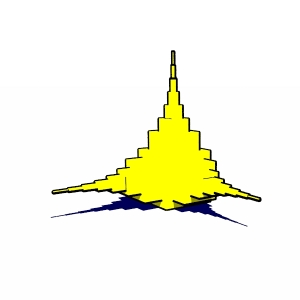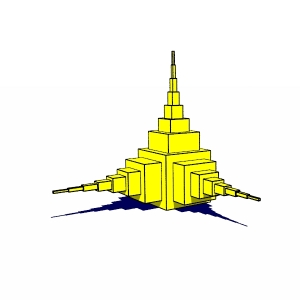PovEdge

A simple tool to draw the edges of your mesh objects in PovRay

Contact :
tuabihtucl at yahoo dot fr

PovEdge computes two different types of edges: Silhouette edges and Crease edges.

1) Silhouette edges give the outline of the object. This of course depends on the point of view on the object, as the outline is the border between the part of the object which is visible, and the part which is hidden. As visible faces have a normal which points towards the viewer, while the hidden faces have a normal which points away from the viewer, PovEdge determines if an edge is part of the silhouette by comparing the relative orientations of the two faces sharing this edge with respect to the vector joining the point of view to the edge. Mathematically, PovEdge computes the scalar product of each normal vector with this vector, and if the signs of these two scalar products are different, then the edge is part of the silhouette.

2) Crease edges show the abrupt changes of orientations in the object (think of the edge of a cube for example). These edges are an intrinsic property of the object, and do not depend on the point of view. To determine if a given edge is part of a crease, PovEdge simply compares the orientation of the two faces sharing this edge. If the orientations of the normals differ by more than a given threshold, then this edge is part of a crease. Mathematically, PovEdge simply compute the scalar product between the two normal vectors, and checks whether it is larger than a given threshold.

Note that, in order to perform these calculations, one needs to know what the two faces which share a given edge are, and this of course for all the edges of the mesh object.  This information, which we call the "edge structure", is not included in the description of the mesh, so it needs to be calculated first. This is what is done by the stand-alone application PovEdge: it parses a given file for all the 'mesh2' declarations, and for each 'mesh2', it computes the edge structure and writes it to a file. Then, to check whether they are part of the silhouette or of a crease, the calculations on the edges are done at parsing time, by a Pov macro (called EdgeMesh).
See the "How to use" section for practical use.Object with silhouette edges only Same object with crease edges